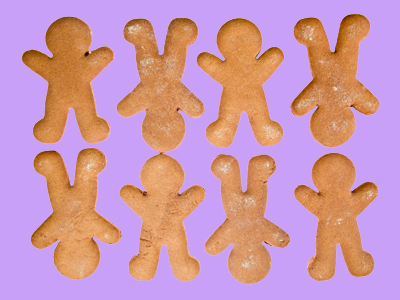2 groups of 4 is the same as 4 groups of 2.

# Year 1 Calculation - Multiplication Using Arrays

This quiz addresses the requirements of the National Curriculum KS1 Maths and Numeracy for children aged 5 and 6 in year 1. Specifically this quiz is aimed at the section dealing with multiplication using arrays.

Multiplication using arrays is a way of organising the numbers in a visual way in order to understand how repeated addition is the same as multiplication. Using an array to visualise a problem also highlights how the order of the multiplication can be reversed to give the same answer. For example, 2 x 4 gives the same answer as 4 x 2.

Question 1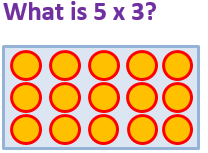30
13
26
15
5 groups of 3, or 3 + 3 + 3 + 3 + 3 = 15
Question 2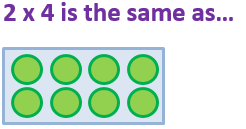2 + 2
4 x 2
4 + 4 + 4
2 x 2
2 groups of 4 is the same as 4 groups of 2
Question 3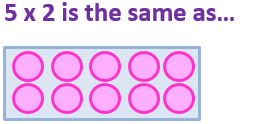2 + 2 + 2 + 2 + 2
4 x 5
5 + 5 + 5 + 5 + 5
5 x 5
5 x 2 is 5 groups of 2, or 2 + 2 + 2 + 2 + 2
Question 4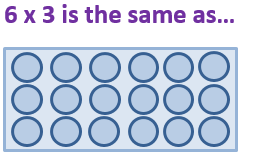3 + 3 + 3 + 3 + 3 + 3
6 + 6 + 6 + 6 + 6 + 6
3 x 3
6 x 6
6 x 3 is the same as 6 groups of 3, or 3 + 3 + 3 + 3 + 3 + 3
Question 5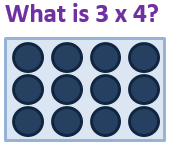18
12
8
14
3 groups of 4, or 4 + 4 + 4 = 12
Question 6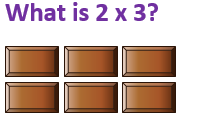6
8
4
3
2 groups of 3, or 3 + 3 = 6
Question 7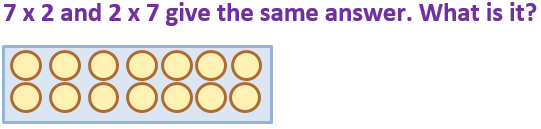72
12
27
14
2 groups of 7, or 7 groups of 2, makes 14
Question 8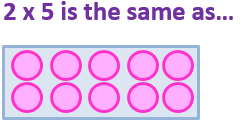2 + 5
4 x 5
5 + 5
5 x 5
2 x 5 is 2 groups of 5, or 5 + 5
Question 9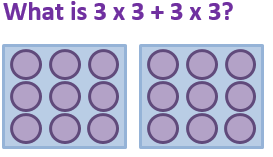18
9
99
81
3 x 3 = 9. 9 plus 9 = 18
Question 10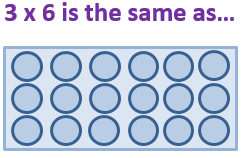3 + 3 + 3
6 + 6 + 6
3 x 3
6 x 6
3 x 6 is the same as 3 groups of 6, or 6 + 6 + 6
You can find more about this topic by visiting BBC Bitesize - How to multiply using an array

Author:  Angela Smith